# Physics 102 Lecture 3 Electric Potential Energy Electric

• Slides: 25Physics 102: Lecture 3 Electric Potential Energy & Electric Potential Physics 102: Lecture 2, Slide 1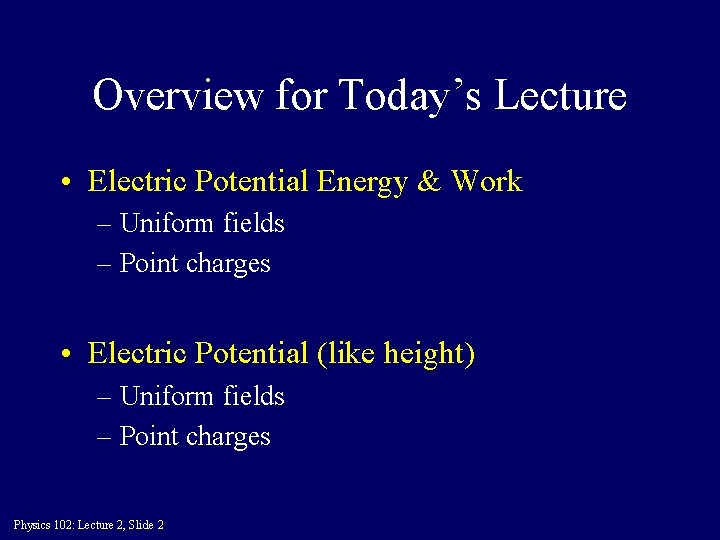Overview for Today’s Lecture • Electric Potential Energy & Work – Uniform fields – Point charges • Electric Potential (like height) – Uniform fields – Point charges Physics 102: Lecture 2, Slide 2Recall Work from Phys 101 • Work done by the force given by: – – W = F d cos(q) Positive: Force is in direction moved Negative: Force is opposite direction moved Zero: Force is perpendicular to direction moved • Careful ask WHAT is doing work! – Opposite sign for work done by you! • Conservative Forces – D Potential Energy = -Wforce Physics 102: Lecture 2, Slide 3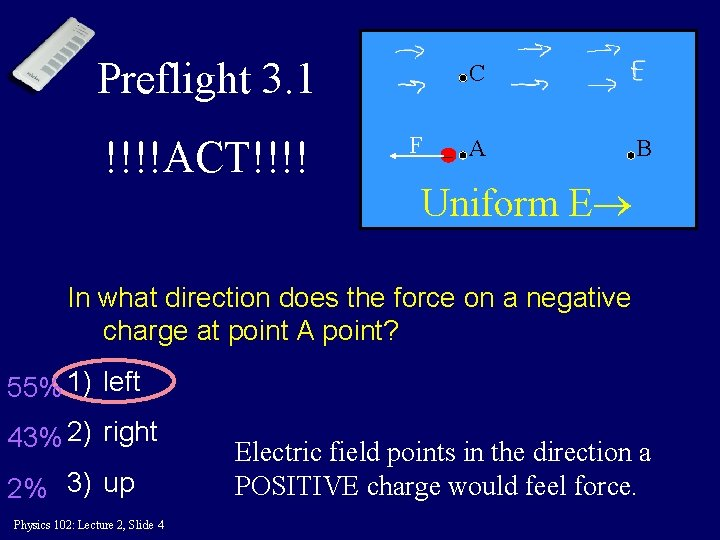Preflight 3. 1 !!!!ACT!!!! C F – A B Uniform E In what direction does the force on a negative charge at point A point? 55% 1) left 43% 2) right 2% 3) up Physics 102: Lecture 2, Slide 4 Electric field points in the direction a POSITIVE charge would feel force.Preflight 3. 2 “I would say zero because the path is perpendicular to the field” motion C F - A B Uniform E When a negative charge is moved from A to C the ELECTRIC force does 8% 1) positive work. 88% 2) zero work. 4% 3) negative work. Physics 102: Lecture 2, Slide 5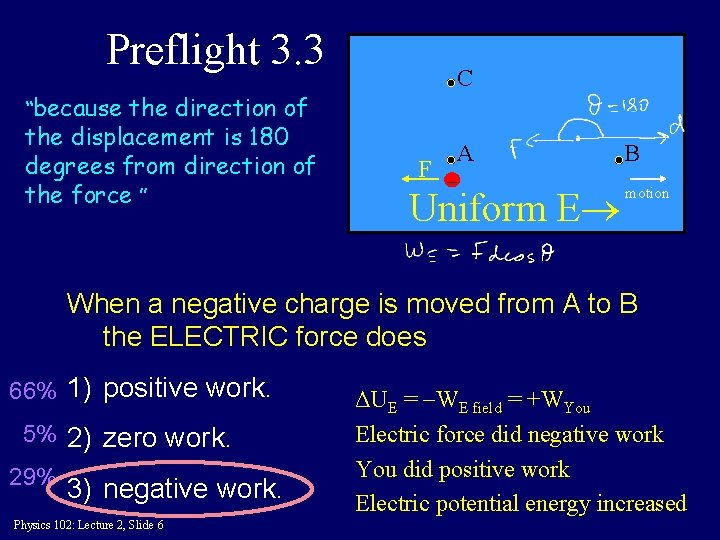Preflight 3. 3 “because the direction of the displacement is 180 degrees from direction of the force ” C A F - Uniform E B motion When a negative charge is moved from A to B the ELECTRIC force does 66% 1) positive work. 5% 2) zero work. 29% 3) negative work. Physics 102: Lecture 2, Slide 6 ΔUE = -WE field = +WYou Electric force did negative work You did positive work Electric potential energy increasedACT: Work WA-B = work done by FE moving charge from A to B C A F - B Uniform E The negative charge is moved from A to C to B. Is the work done by the electric force: A) Greater than WA-B B) Same as WA-B C) Less than WA-B Physics 102: Lecture 2, Slide 7 Path does not matter! Only end points matter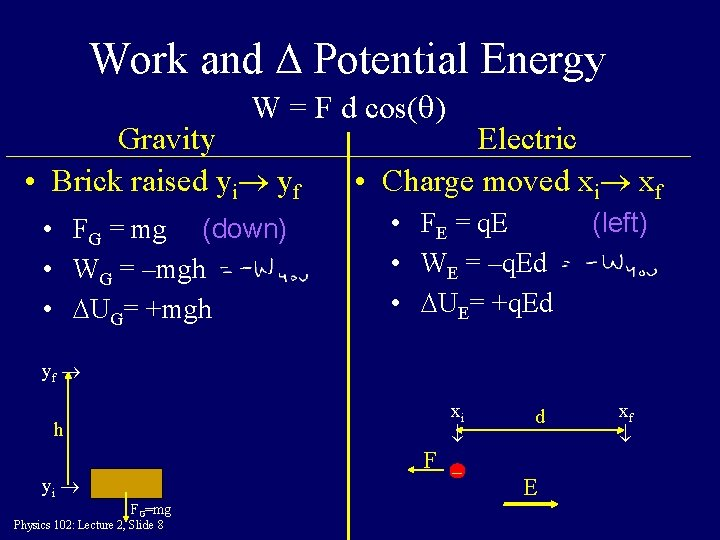Work and D Potential Energy W = F d cos(q) Gravity • Brick raised yi yf • FG = mg (down) • WG = –mgh • DUG= +mgh Electric • Charge moved xi xf • FE = q. E • WE = –q. Ed • DUE= +q. Ed (left) yf xi h F - yi FG=mg Physics 102: Lecture 2, Slide 8 d E xfE. P. E. for point charges E. P. E. of two charges q 1 and q 2 separated a distance r: What is the electric potential energy of an electron a distance r = 0. 53 10 -10 m from a proton (H atom)? UE = (+1. 6 10 -19)(-1. 6 10 -19)/0. 53 10 -10 = -4. 35 10 -18 J rf = 0. 5 10 -10 m + Physics 102: Lecture 2, Slide 9 -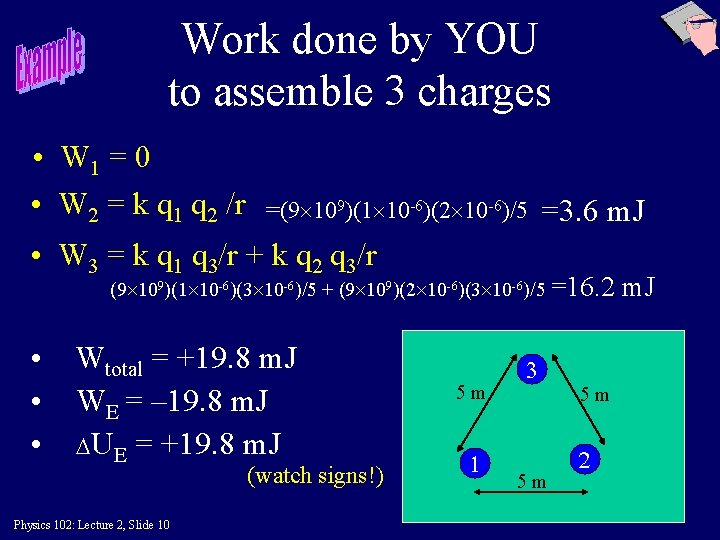Work done by YOU to assemble 3 charges • W 1 = 0 • W 2 = k q 1 q 2 /r =(9 109)(1 10 -6)(2 10 -6)/5 =3. 6 m. J • W 3 = k q 1 q 3/r + k q 2 q 3/r (9 109)(1 10 -6)(3 10 -6)/5 + (9 109)(2 10 -6)(3 10 -6)/5 =16. 2 • • • Wtotal = +19. 8 m. J WE = – 19. 8 m. J DUE = +19. 8 m. J (watch signs!) Physics 102: Lecture 2, Slide 10 5 m 1 3 5 m 5 m 2 m. J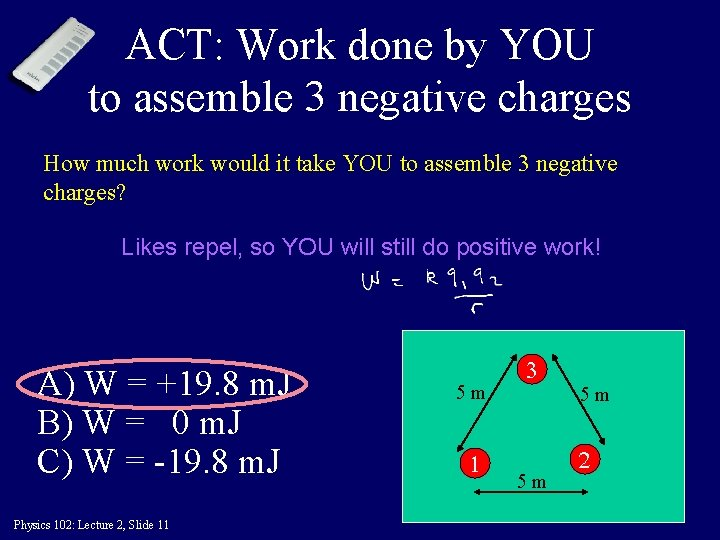ACT: Work done by YOU to assemble 3 negative charges How much work would it take YOU to assemble 3 negative charges? Likes repel, so YOU will still do positive work! A) W = +19. 8 m. J B) W = 0 m. J C) W = -19. 8 m. J Physics 102: Lecture 2, Slide 11 5 m 1 3 5 m 5 m 2Preflight 3. 11 1 5 m 2 + + 5 m 5 m - 3 The total work required by you to assemble this set of charges is: 50% (1) positive Bring in (1): zero work 18% (2) zero Bring in (2): positive work 32% (3) Physics 102: Lecture 2, Slide 12 negative Bring in (3): negative work x 2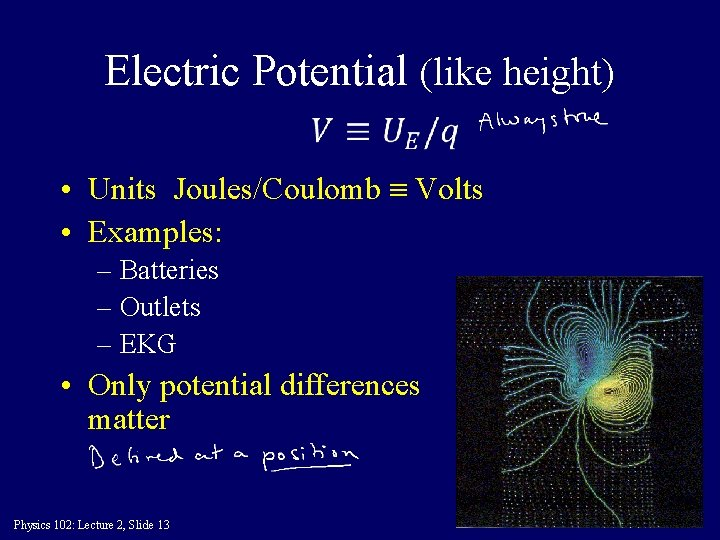Electric Potential (like height) • Units Joules/Coulomb Volts • Examples: – Batteries – Outlets – EKG • Only potential differences matter Physics 102: Lecture 2, Slide 13Electric Potential (like height) Devil’s Tower Topographical map Moving to higher potential moving uphill Physics 102: Lecture 2, Slide 14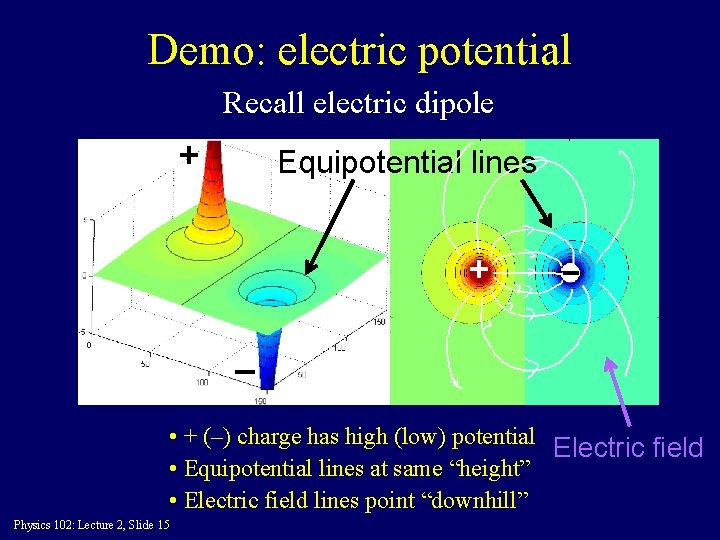Demo: electric potential Recall electric dipole + Equipotential lines + – – • + (–) charge has high (low) potential Electric field • Equipotential lines at same “height” • Electric field lines point “downhill” Physics 102: Lecture 2, Slide 15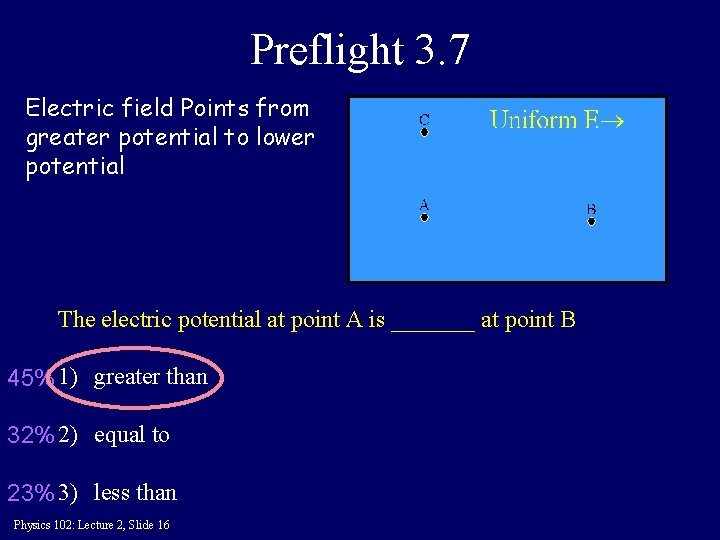Preflight 3. 7 Electric field Points from greater potential to lower potential The electric potential at point A is _______ at point B 45% 1) greater than 32% 2) equal to 23% 3) less than Physics 102: Lecture 2, Slide 16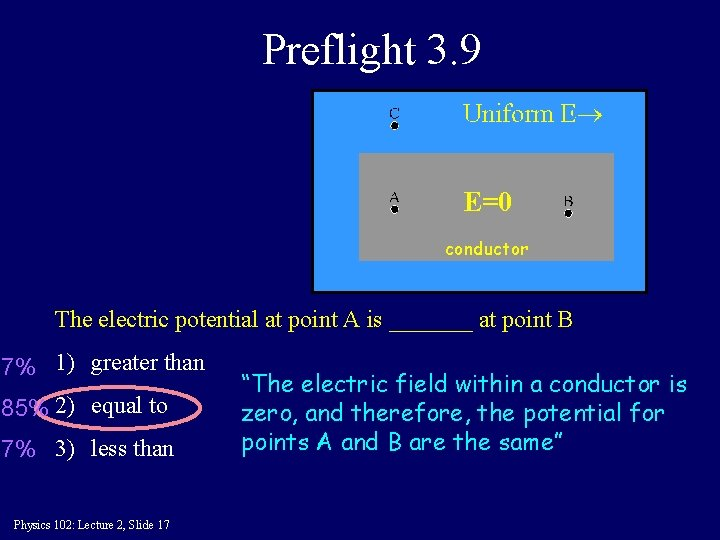Preflight 3. 9 E=0 conductor The electric potential at point A is _______ at point B 7% 1) greater than 85% 2) equal to 7% 3) less than Physics 102: Lecture 2, Slide 17 “The electric field within a conductor is zero, and therefore, the potential for points A and B are the same”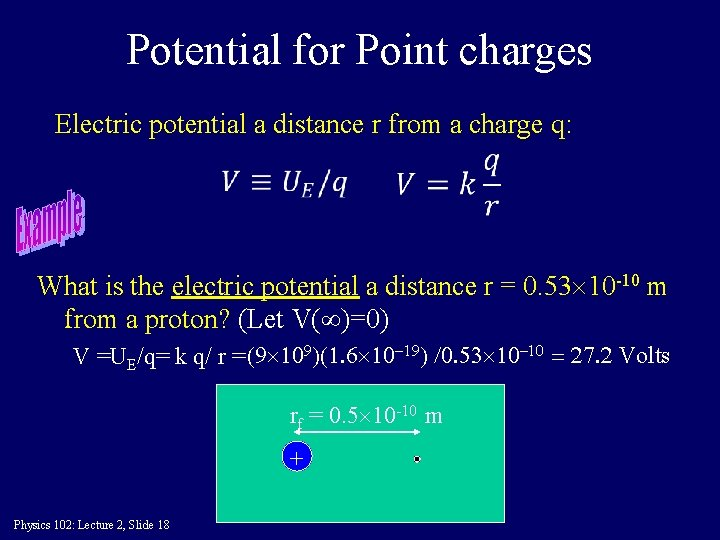Potential for Point charges Electric potential a distance r from a charge q: What is the electric potential a distance r = 0. 53 10 -10 m from a proton? (Let V( )=0) V =UE/q= k q/ r = (9 109)(1. 6 10 -19) /0. 53 10 -10 = 27. 2 Volts rf = 0. 5 10 -10 m + Physics 102: Lecture 2, Slide 18Two Charges • Calculate electric potential at point A due to charges – Calculate V from +7 m. C charge – Calculate V from – 3. 5 m. C charge – Add (EASY! NO VECTORS) A 4 m V = kq/r V 7 = (9 109)(7 10 -6)/5 = 12. 6 103 V V 3 = (9 109)(-3. 5 10 -6)/5 = -6. 3 103 V Vtotal = V 7+V 3 = +6. 3 103 V Q=+7. 0 m. C How much work do you have to do to bring a 2 m. C charge from far away to point A? Physics 102: Lecture 2, Slide 19 6 m Q=-3. 5 m. C W=DU=Vq = (+6. 3 103 V)(2 m. C) = +12. 6 m. JACT: Two Charges • In the region II (between the two charges) the electric potential is 1) always positive 2) positive at some points, negative at others. 3) always negative I II Q=+7. 0 m. C III Q=-3. 5 m. C Very close to positive charge potential is positive Very close to negative charge potential is negative Physics 102: Lecture 2, Slide 20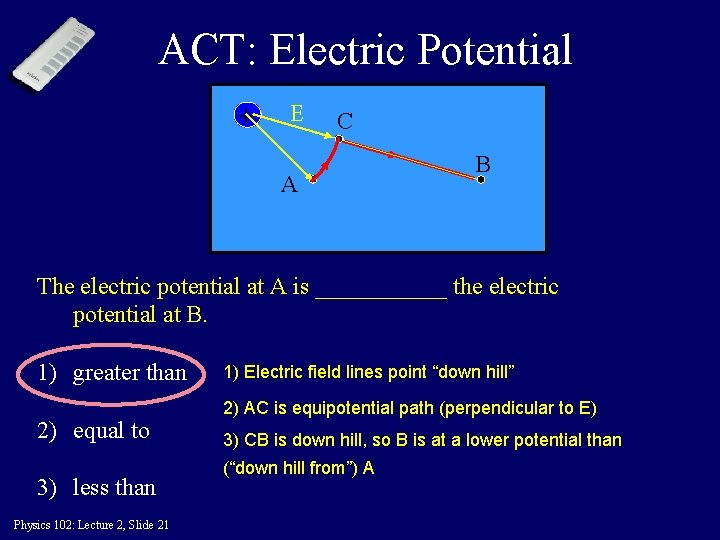ACT: Electric Potential + E C A B The electric potential at A is ______ the electric potential at B. 1) greater than 2) equal to 3) less than Physics 102: Lecture 2, Slide 21 1) Electric field lines point “down hill” 2) AC is equipotential path (perpendicular to E) 3) CB is down hill, so B is at a lower potential than (“down hill from”) AComparison: Electric Potential Energy vs. Electric Potential • Electric Potential Energy (U) - the energy of a charge at some location. • Electric Potential (V) - found for a location only – tells what the EPE would be if a charge were located there (usually talk about potential differences between two locations): U = Vq • Neither has direction, just value. Sign matters! Physics 102: Lecture 2, Slide 22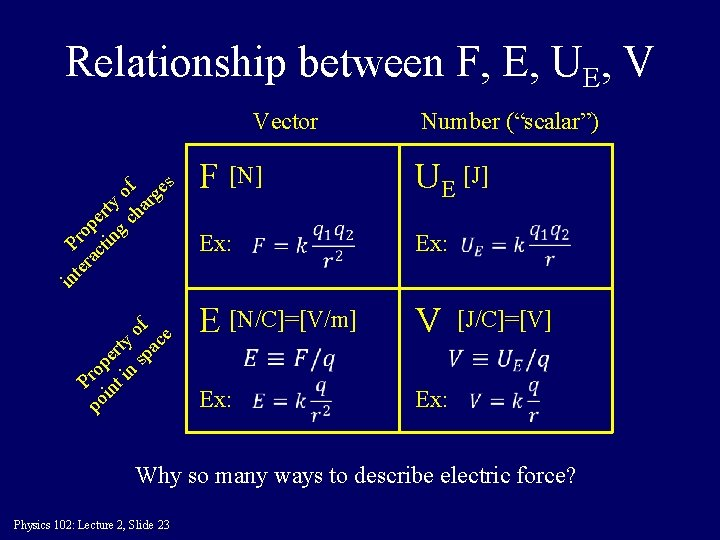Relationship between F, E, UE, V Number (“scalar”) F [N] UE [J] Ex: E [N/C]=[V/m] V Ex: po Prop in er t i ty n sp of ac e in te Pro ra p ct er in ty g ch of ar ge s Vector [J/C]=[V] Why so many ways to describe electric force? Physics 102: Lecture 2, Slide 23Electron microscope ΔV=10 k. V Vi - Vf Electron gun Uniform E motion • What is the final velocity of the e-? • Solve by conservation of energy: K. E. i + P. E. i = K. E. f + P. E. f 0 + –e. Vi = ½mv 2 + –e. Vf Physics 102: Lecture 2, Slide 24 Could solve this using F=ma & kinematic equations (Phys 101) TRY AT HOME! (HARDER)See everyone Monday! Physics 102: Lecture 2, Slide 25